## research papersJOURNAL OFSYNCHROTRONRADIATION
ISSN: 1600-5775
Volume 25| Part 3| May 2018| Pages 650-654

## XFEL experiments: jitter of pump–probe time delays and pulse intensities

aSorbonne Universités, UPMC Université Paris 06, Laboratoire Physique Théorique de la Matière Condensée, 75005 Paris, France, and bESRF – The European Synchrotron, Complex Systems and Biomedical Sciences (CBS), CS 40220, 38043 Grenoble Cédex 9, France
*Correspondence e-mail: bratos@lptmc.jussieu.fr

(Received 16 February 2017; accepted 1 March 2018; online 28 March 2018)

Jitter of XFEL signals due to fluctuations in shot-to-shot time delays and intensities are explored in the frame of a statistical theory of X-ray diffraction from liquids. Deformed signals are calculated at different levels of pump–probe jitter. A new method is proposed to eliminate these distortions.

### 1. Introduction

Monitoring atomic motions during a chemical reaction has always been an important objective in chemical research. This sort of `filming', inaccessible in the past, can now be realized either by performing time-resolved optical or time-resolved X-ray experiments. Optical experiments, less expensive than X-ray experiments, were realized first and proved to be highly efficient. The Nobel prize for chemistry was awarded to A. Zewail for his spectacular achievements in this field (Zewail, 2000). However, as the wavelengths of optical waves are large compared with inter-atomic distances in molecules, optical techniques cannot detect atomic positions without complementary assumptions. This difficulty is absent in X-ray experiments. These experiments can be realized both in diffraction or absorption using either synchrotron or free-electron laser (XFEL) techniques. Pulses of the order of 100 ps can be generated by the former and 10 fs by the latter. X-ray techniques, in particular XFEL techniques, have proven to be extremely efficient, but difficulties still limit, for the time being, their intrinsic power, i.e. the shot-to-shot dispersion of pump–probe time delays and of pulse intensities. Important efforts has been made to solve this problem experimentally (Meyer et al., 2006; Fritz et al., 2007; Maltezopoulos et al., 2008; Azima et al., 2009; Glownia et al., 2010; Löhl et al., 2010; Bionta et al., 2011; Tavella et al., 2011). The recent measure and sort technique (Harmand et al., 2013) merits attention in this context. We complete this effort theoretically by calculating the signal distortions in some typical situations. We also propose a new method to eliminate these distortions.

### 2. Theory

In a time-resolved X-ray experiment, the sample is pumped by an optical pulse and probed by an X-ray pulse. The pump–probe time delay must be determined with extreme accuracy. At the present time, while XFEL sources generate pulses down to 10 fs, there is a jitter on the pump–probe time delays of several hundred femtoseconds. The experiment must thus be repeated and the resulting signals averaged over this sequence to make the results usable. In this way, a single-pulse experiment transforms into a multi-pulse experiment. The problem is thus statistical, not only in its molecular dynamics part but also in the electric field part. Statistical mechanics are thus omnipresent, as in ultrafast optical spectroscopy; see, for example, Mukamel (1995).

A statistical theory of X-ray diffraction from liquids was published some time ago (Bratos et al., 2002). Its full mathematical development is given in this reference, and will not be repeated again. Only the essential features are illustrated in the following. The intensity of the diffracted X-rays, ΔS(q, τ), is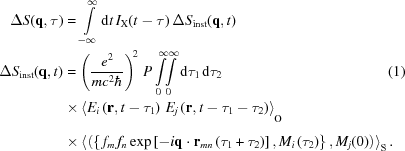Here, P is a factor characteristic of the experimental set-up such as the temporal pulse profile, polarization, sample concentration, etc. IX is the intensity of the incident X-ray radiation, Ei, Ej are components of the electric field generated by the optical laser, q is the wavevector, fm, fn are atomic scattering factors, rmn is the distance between the atoms m and n, and Mi, Mj are components of the laser-induced transition moment M between the states i and j. Einstein's convention of summing over doubled indices i,j and m,n is employed. The form of this expression can be understood comparing it with the standard expression for the diffracted X-ray intensity S(q) ≃ Σm,n[fmfnexp(−iq·rmn)] (Warren, 1990). The latter is valid if the incident X-ray wave has a constant amplitude and if fast chemical processes are absent. If the incident X-ray beam consists of short pulses, and if some fast chemical process is laser excited, this expression must be modified in two ways. First, the intensity and the inter-atomic distances rmn are now time dependent, and IX and rmn must be replaced by IX(t) and rmn(t). The remaining quantities in equation (1)describe the laser-induced electronic excitation. This can be understood by noticing that, according to the Fermi golden rule, the rate of this excitation is proportional to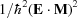, where E is the laser-generated electric field and M is the transition moment. The presence in equation (1)of the factors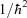, E(tτ1), E(tτ1τ2), M(0) and M(τ2) can be understood in this way. The connection of different time points cannot be explained as simply. This equation can be used as it stands when studying single-pulse events.

Interpreting multi-pulse experiments is more complex, due to the scatter of pump–probe time delays and shot intensities. However, the form of equation (1)indicates that these problems can be studied independently from those due to molecular dynamics. Note also that equation (1)was conceived for a single-pulse experiment. However, a slight modification makes it applicable to a multi-pulse experiment: it is sufficient to replace the single X-ray pulse intensity IX(tτ) by the average multi-pulse intensity 〈IX(tτ)〉MP, the index MP indicating multi-pulse. One can then write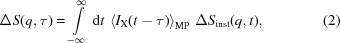where ΔSinst(q, t) is the same as in equation (1). In the rest of this paper, the incident X-ray beam is supposed to be Gaussian,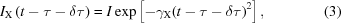where τ is the nominal pump–probe time delay, δτ its ill-controlled shot-to-shot time increment and (1/γX)1/2 its temporal width.

To proceed further, details about the statistical distribution of δτ and I for subsequent shots are required. The attention of the experimentalists was centered on this for years, and still remains an issue. According to the literature (Cavalieri et al., 2005), the distribution of pump–probe time delays P(δτ) is Gaussian: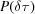=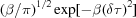. The distribution of shot-to shot intensities P(I) is less well known, but according to equation (2)it is needed only if the absolute intensity of the scattered radiation is explored, which is not the case here. Then, inserting (3)into (2)and integrating over δτ results in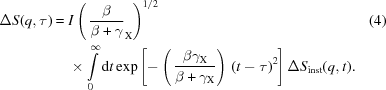Jitter thus generates an effective temporal broadening of incident X-ray pulses. This is the basic equation relating the distorted and non-distorted signals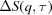and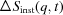, respectively.

To proceed further, the following method can be chosen. Let the laser excitation promote the molecules from their ground electronic state 0, where the length of a given bond is r0, to an electronic state 1, where it is r1. According to the Franck–Condon principle, r1(0) = r0 at time t = 0. The simplest assumption to describe the bond length variation at later times consists of writing r(t) =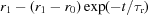, where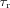is the molecular reaction (or rearrangement) time (Fig. 1). The signal, not affected by pump–probe time delay dispersion, can be written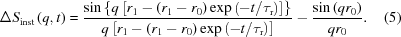Then, inserting (5)into (4)and integrating provides. The integration can be performed either numerically or analytically if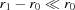. Note that this condition is not very restrictive. When passing from a single C—C bond to a triple C≡C bond, r0 = 1.5 Å and r0r1 = 0.3 Å. The experimental signalcan then be calculated and its distortion investigated, if the parameters r1 andare known. The opposite problem of extracting the non-perturbed signalfrom the observed signalis more difficult. It is best to work with the functionin its analytical form,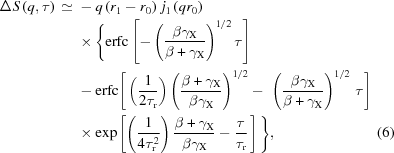where r0 and r1 are bond lengths before and after reaction, τr is its characteristic time and j1(x) is the Bessel function of order 1 [remember that sinx/x is the Bessel function j0(x)]. Inserting experimental data into the left-hand side of (6)then permits calculation of r1 and τr using mean square optimization techniques. As there are only two parameters r1 and τr to determine, this calculation is easy.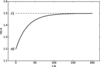Figure 1 Variation of the bond length r(t) from r0 to the laser-excited state r1.

The corresponding r-space signals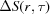can be calculated by Fourier inverting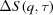. This can be done without any special precaution if τ is large compared with the time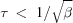characteristic of pump–probe dispersion. If this is not the case,must be corrected by carrying out the above procedure for each q,τ point such that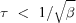, making the Fourier transform possible. It is thus more difficult to correct the signals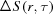than the signals.

### 3. Examples

#### 3.1. Times shorter than the molecular dynamics

The times considered here are of the order of 10 fs or shorter. At these times a liquid behaves like a glass. Nevertheless, diffraction signals still vary with time, even if all inter-atomic distances r are fixed. This is due to the electric fields Ei, Ej of the optical pump pulses in equation (1). The noise of XFEL radiation also plays a major role. In this limit, one finds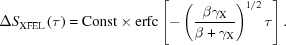One concludes that the dispersion of pump–probe time delays modifies the temporal width of the average multi-pulse signals even at very short times. These effects may be large, even overwhelmingly large (compare with Fig. 2). Note also that in this short-time limit the q- and r-resolved signals exhibit the same τ dependence. In fact, in this limit,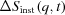is independent of time. A look at equation (6)then confirms the statement.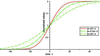Figure 2 Variation of ΔS at the shortest pump–probe times delays.

#### 3.2. Contracting chemical bond

In the absence of distortion-free experimental data in the 10–100 fs time domain, the following example is completely theoretical. Let us start by considering a C–C bond contracting from 1.5 Å to 1.2 Å; these values correspond to a single and triple C–C bond, respectively. This C–C bond is supposed to be a part of a polyatomic molecule PolyM. Its C–C diffraction peak is assumed to be sufficiently isolated from other diffraction peaks from PolyM to be explorable. The laser pump promotes PolyM from its electronic ground state A, where the C–C bond is simple, to a state B, where it is triple. However, this transformation is not instantaneous: according to the Franck–Condon principle, light-induced transitions are all vertical. At τ = 0, the C–C distance remains unchanged, equal to 1.5 Å. It is only at later times that it contracts gradually from 1.5 Å to 1.2 Å. How does this contraction process manifests itself in an r-resolved XFEL experiment? And how does this signal deform if the pump–probe times are dispersed? The central quantities are the pair distribution functions g(r,t) (see Hansen & MacDonald, 2006). The following expressions are chosen in our model,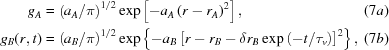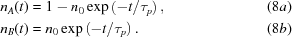Here, τp is the population relaxation time. Note that gA(r) and gB(r,t) approach a δ function when aA and aB go to infinity. Equation (7b)states that the C–C bond contracts in the state B of PolyM in times of the order of τv. Employing the above equations together with equations (2)and (4)generates the r-resolved signal ΔS(r,τ). The parameters of the above model are: the ground state distance rA is 1.5 Å and the excited states distance rB is 1.2 Å; the laser-induced contraction of the C–C bond in the state B of PolyM is 0.3 Å. The parameters aA and aB are both of the order of 25 Å−2, which corresponds to a half-width of gA(r) and gB(r,t) of the order of 0.4 Å. Moreover, the recombination time τv is assumed to be of the order of 100 fs, and τp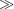τ. These values correspond to an ultrafast chemical process.

Fig. 3(a)illustrates ΔS(r,τ), the r-resolved XFEL signal of a contracting C–C bond in the absence of pump–probe time dispersion. This signal is presented in three dimensions: the distance r and the time τ are defined on the two coordinate axes while the intensity is given by the color. The red valley at 1.5 Å pictures the deficit of C–C bonds at the initial bond length of 1.5 Å, whereas the violet ridge indicates C–C bonds of given length r at a given time τ. Note that the intensity of the differential signal is vanishing at τ = 0: according to the Frank–Condon principle, electronic transitions are vertical. At times τ ≃ 10 fs, intramolecular dynamics of PolyM intervene noticeably. It is only at times τ > 20 fs that chemistry manifests itself predominantly. The signal represents a film of a contracting C–C bond. If pump–probe times are dispersed, the above picture is slightly or deeply modified; see Fig. 3(b)where ΔS(r,τ) is only blurred. It is only blurred if the pump–probe time dispersion is small. If the pump–probe dispersion time is not small, the C–C contraction is no longer observable and only an instantaneous jump between the initial and final configurations is observed. This effect is widely known in other fields of physics and chemistry under the name of motional narrowing.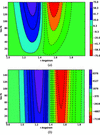Figure 3 Contraction of the C–C bond after laser excitation: the multi-pulse signal in r space. The C–C bond contracts from the single bond length (1.5 Å) to the triple bond length (1.2 Å). The process is supposed to be accomplished in 100 fs. (a) The signal ΔS(r,τ) in the absence of pump–probe time delay dispersion and (b) in its presence (1000 fs). The contraction of the C–C bond is clearly visible in (a) but not in (b).

Moving on from the r-resolved signals ΔS(r,τ), let us now discuss the q-resolved signals ΔS(q,τ). The latter can be deduced from ΔS(r,τ) using the well known formula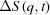=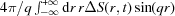, which, according to the basic theory of X-ray diffraction, relates r-resolved and q-resolved signals (Warren, 1990). It is valid independently of whether pump–probe time delays are dispersed or not. This integration was accomplished numerically. The results are presented in Fig. 4. In Fig. 4(a)the signal is calculated for β = infinity, i.e. in the absence of pump–probe dispersion. It is presented in three dimensions: the variables q and τ are placed on the coordinate axes, whereas the value of the signal ΔS(q,τ) is indicated by the color. Violet crests indicate an increase of the signal intensity and red valleys indicate a decrease. The bending of the red crests toward large q values indicates progressive C–C contraction from 1.5 Å to 1.2 Å. This signal is strictly vanishing at τ = 0, whatever the value of q, which is a consequence of the Franck–Condon principle. At long times, ΔS(q,τ) approaches the limit const[r1 2sin(qr1)/qr1-r0 2sin(qr0)/qr0] (Fig. 4b). Atomic motions during a chemical reaction can thus be monitored in this way. Nevertheless, visualizing atomic motions is much more difficult in q-resolved than in r-resolved signals. Fig. 4(b)pictures this signal in the presence of appreciable pump–probe time dispersion. Only immutable red and violet crests are now visible; molecular dynamics can no longer be followed. Motional narrowing is dominating.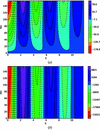Figure 4 Contraction of the C–C bond after laser excitation: the multi-pulse signal in q space. This signal is defined as the difference of multi-pulse signals S(q,τ) in the presence or absence of pump–probe time delay dispersion. Time-delay dispersion is supposed to be of the order of 1000 fs. The contraction is no longer perceptible at this level of jitter.

### 4. Conclusion

Fluctuations of a multi-pulse signal due to shot-to-shot variations in time delays and intensities are explored theoretically in the frame of a statistical theory of X-ray diffraction of liquids. A new method is also proposed to eliminate the effect of time delay jitter in XFEL experiments. Contrary to the measure and sort method which is fully experimental, the present method belongs to the ensemble of signal treatment methods. It does not require any extra experiment.

### ReferencesJOURNAL OFSYNCHROTRONRADIATION